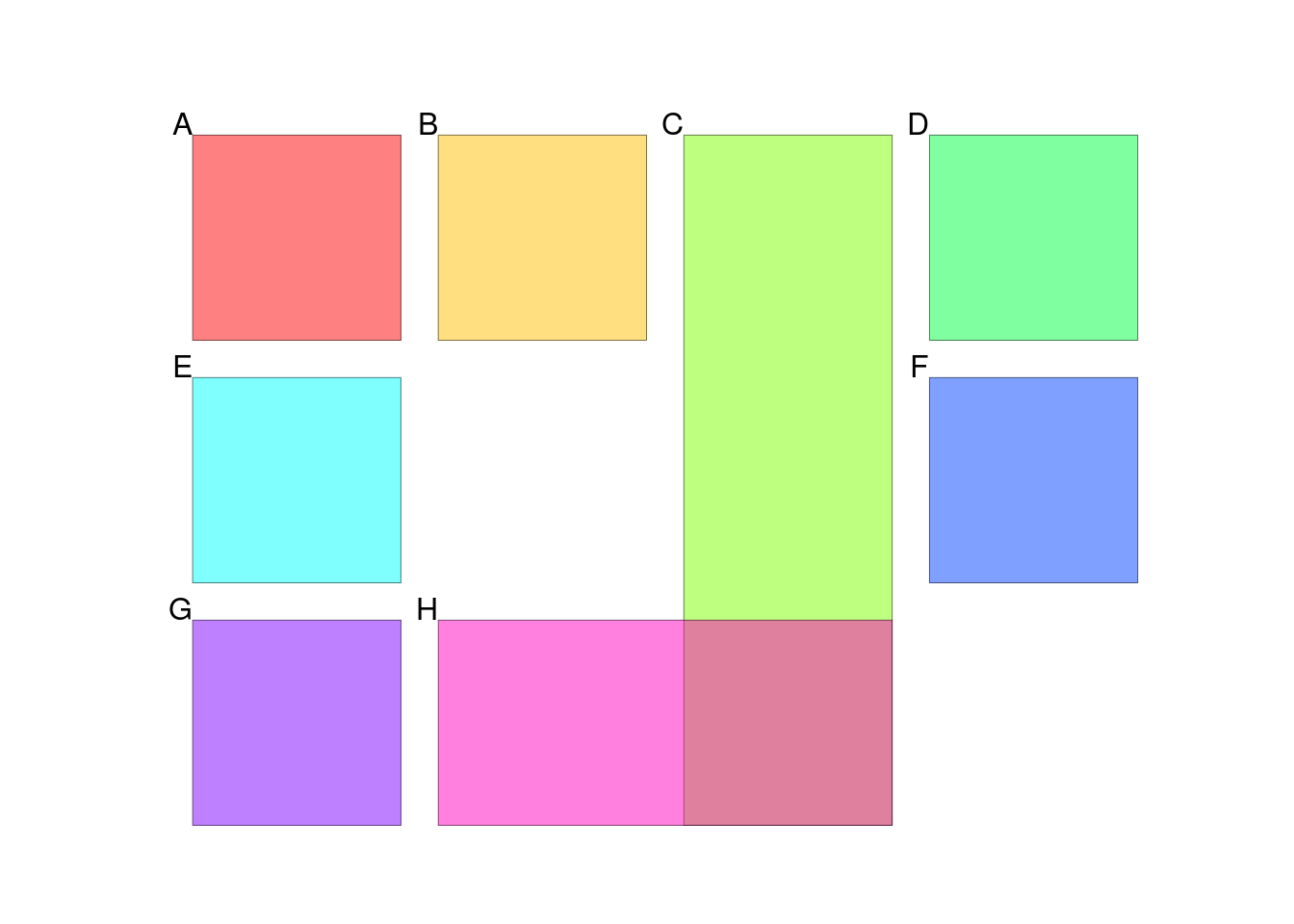When you add a plot or image to a multi-panel figure, you can specify which panels it should cover. The fill_panel function will automatically choose the next free location for you if you don’t specify it.

Here is a multi-panel figure with twelve panels.

library(multipanelfigure)
library(grid)
cols <- 4
rows <- 3
figure <- multi_panel_figure(
width = 135,
columns = cols,
height = 100,
rows = rows)

We will fill the panels with colored rectangle. The next function is designed to change the color of the rectangle each time it is called. Don’t worry too much about its implementation.

rect_grob <- function()
{
this_env <- environment(rect_grob)
assign("current", this_env$current + 1, this_env) rectGrob(gp = gpar(fill = rainbow(8)[this_env$current], alpha = 0.5))
}
assign("current", 0, environment(rect_grob))

If we don’t specify which panel to fill, fill_panel defaults to using the top-left panel.

(figure %<>% fill_panel(rect_grob()))
## Setting row to 1
## Setting column to 1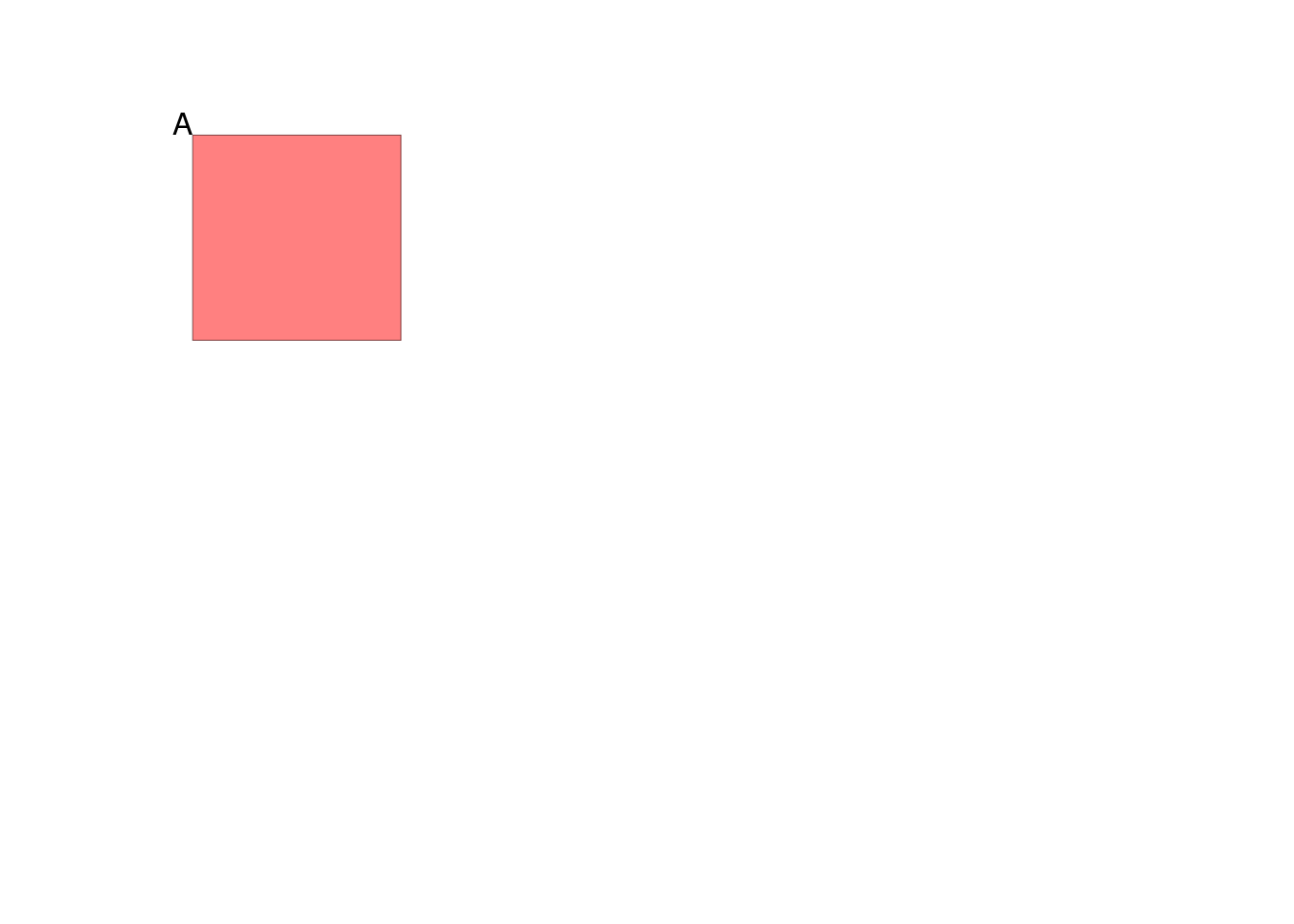Next, the automatically chosen position is the second column on the top row. In general, the default position moves left to right, then top to bottom – just as you would read in most Roman, Greek, or Cyrillic scripts.

(figure %<>% fill_panel(rect_grob()))
## Setting row to 1
## Setting column to 2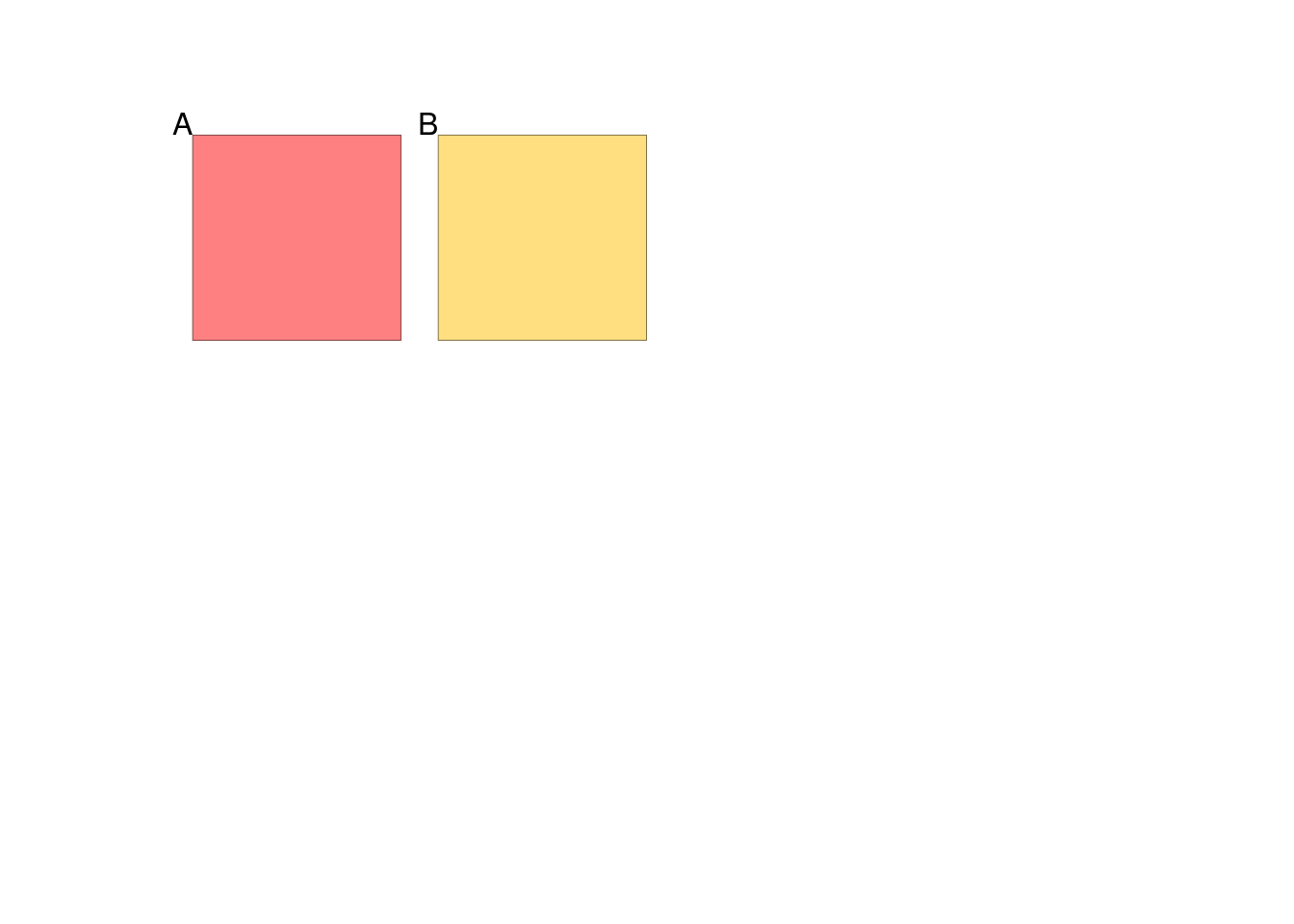If you want to cover multiple panels, or specify a different position, you can manually set the row and column arguments. In the following example, row is set so that the rectangle covers rows one to three. The column argument specifies that the third column should be used; as it is of length one, the image only covers a single column.

(figure %<>% fill_panel(rect_grob(), row = 1:3, column = 3))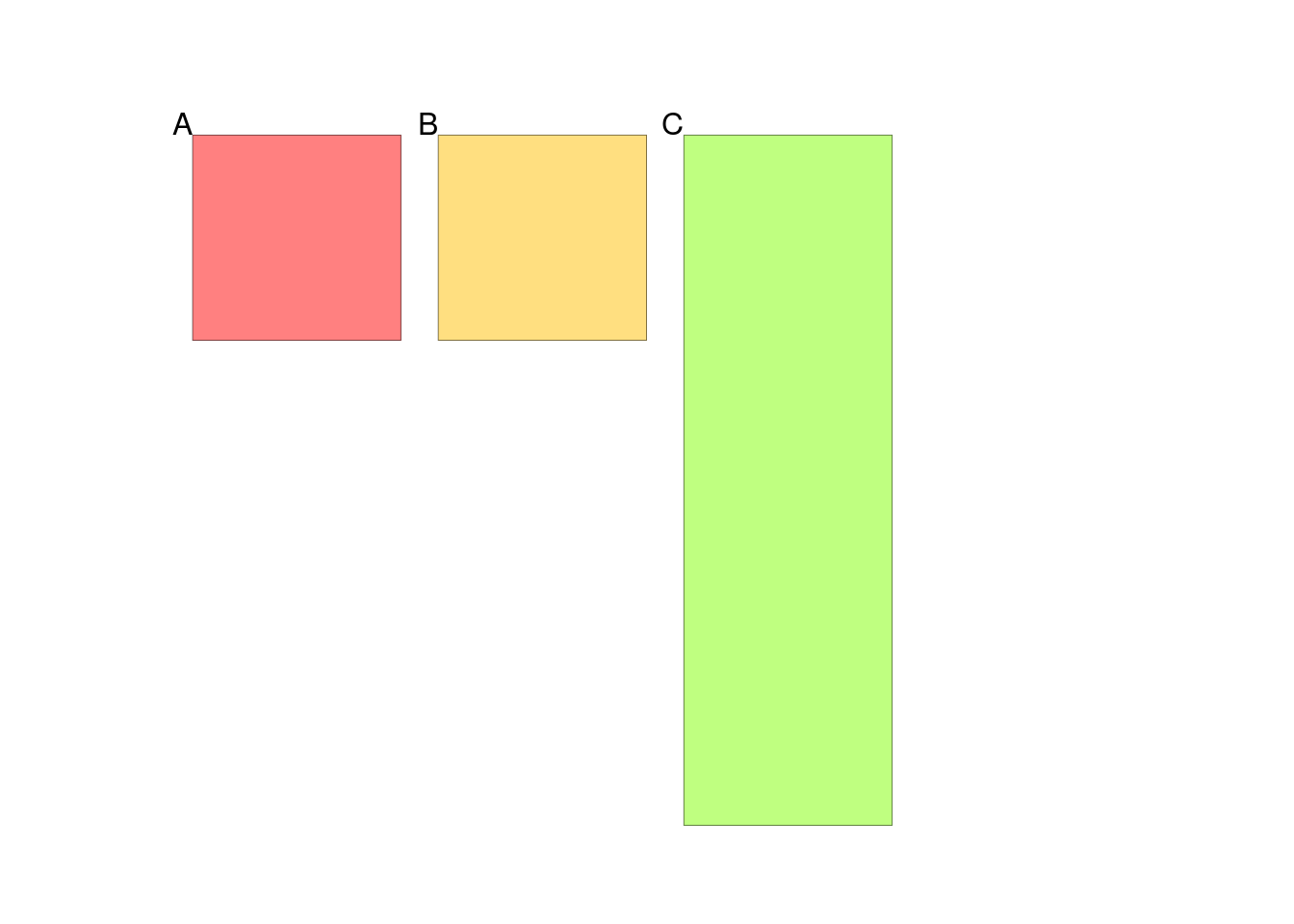Now that the third column in the top row has been filled, the automatic positioning fills the fourth column in the top row.

(figure %<>% fill_panel(rect_grob()))
## Setting row to 1
## Setting column to 4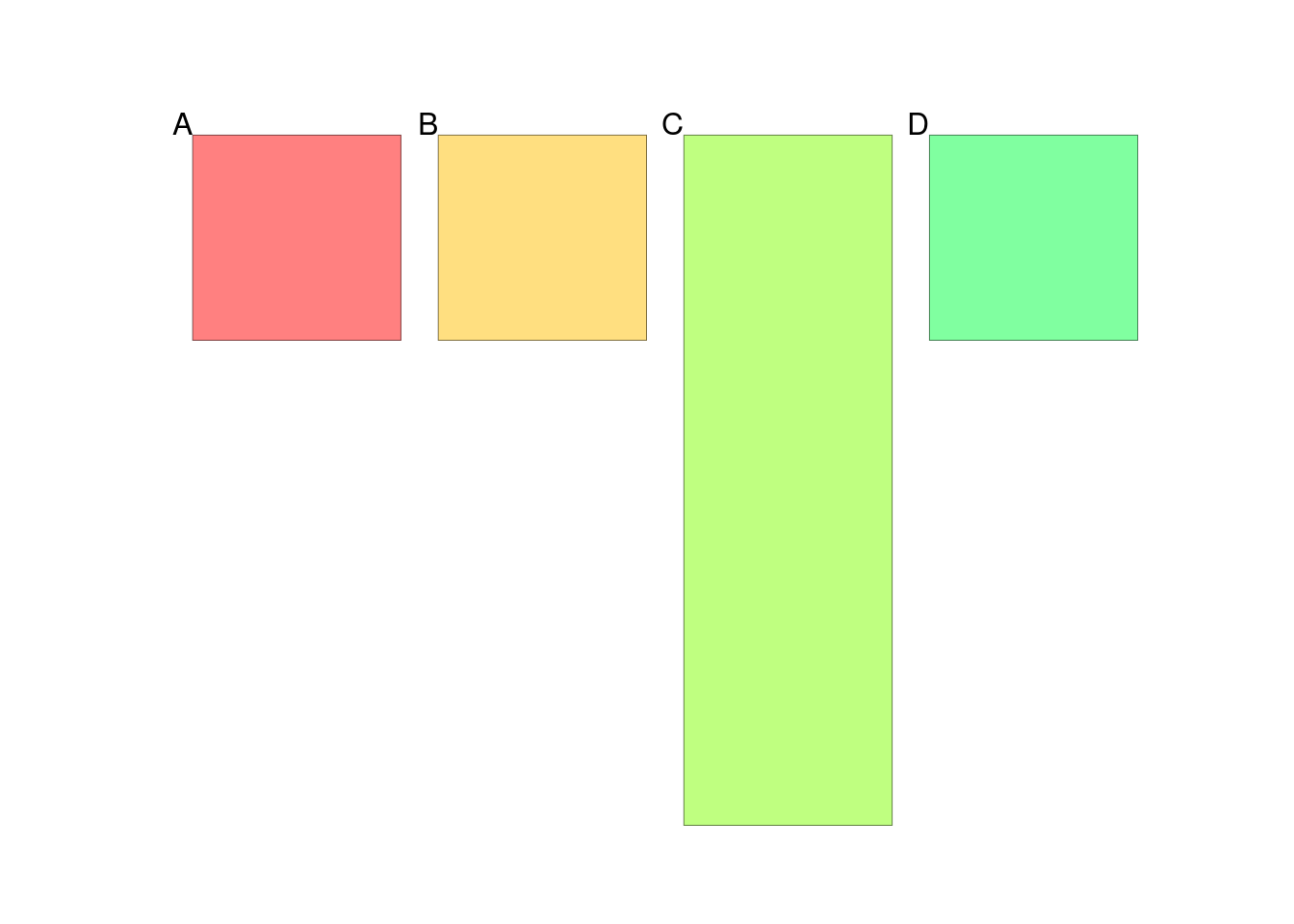The top row is now completely full, so automatic positioning fills the first value in the second row.

(figure %<>% fill_panel(rect_grob()))
## Setting row to 2
## Setting column to 1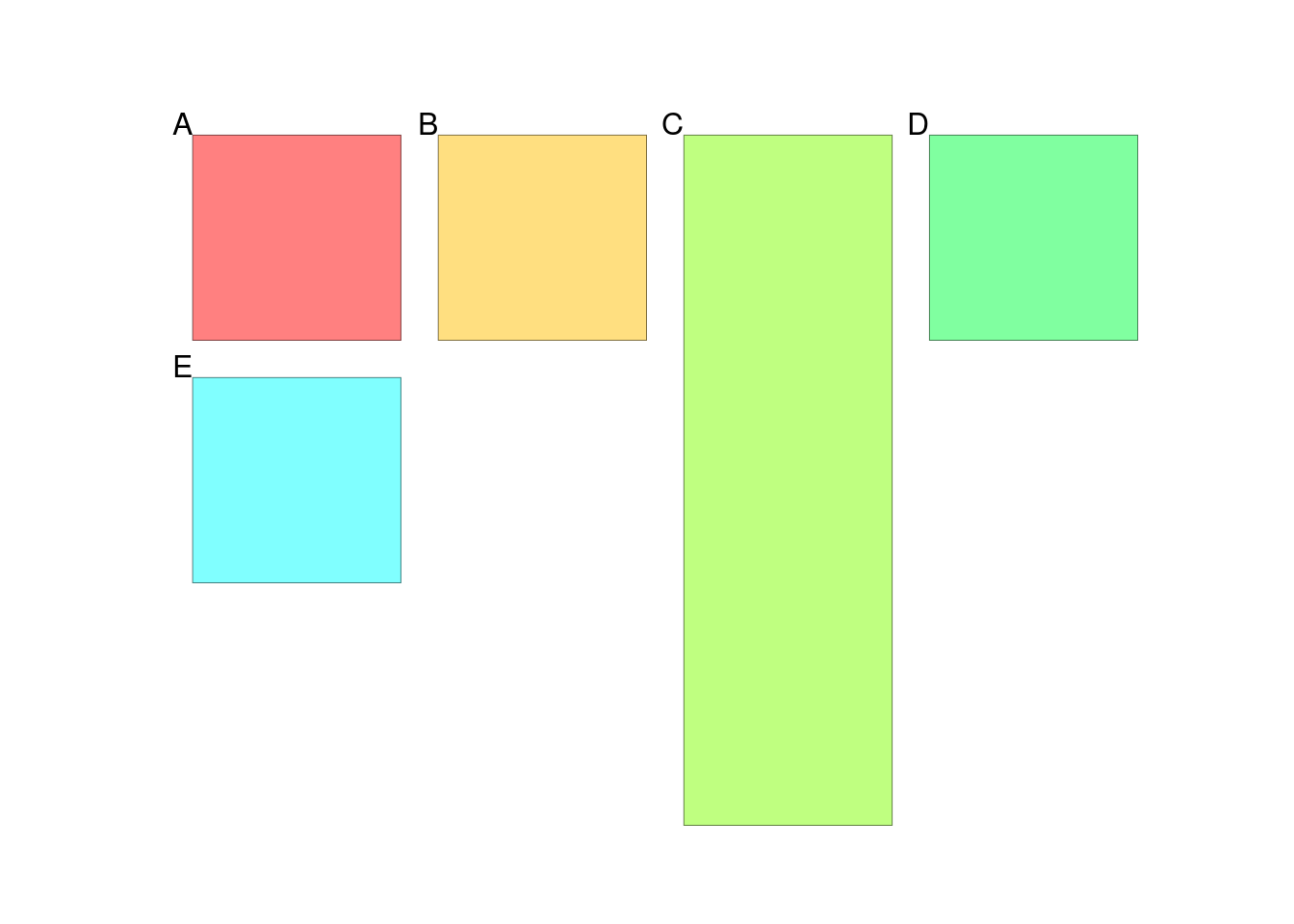Automatic positioning works independently for the row and the column. Here we use automatic positioning for the row but specify the fourth column using left_panel.

(figure %<>% fill_panel(rect_grob(), column = 4))
## Setting row to 2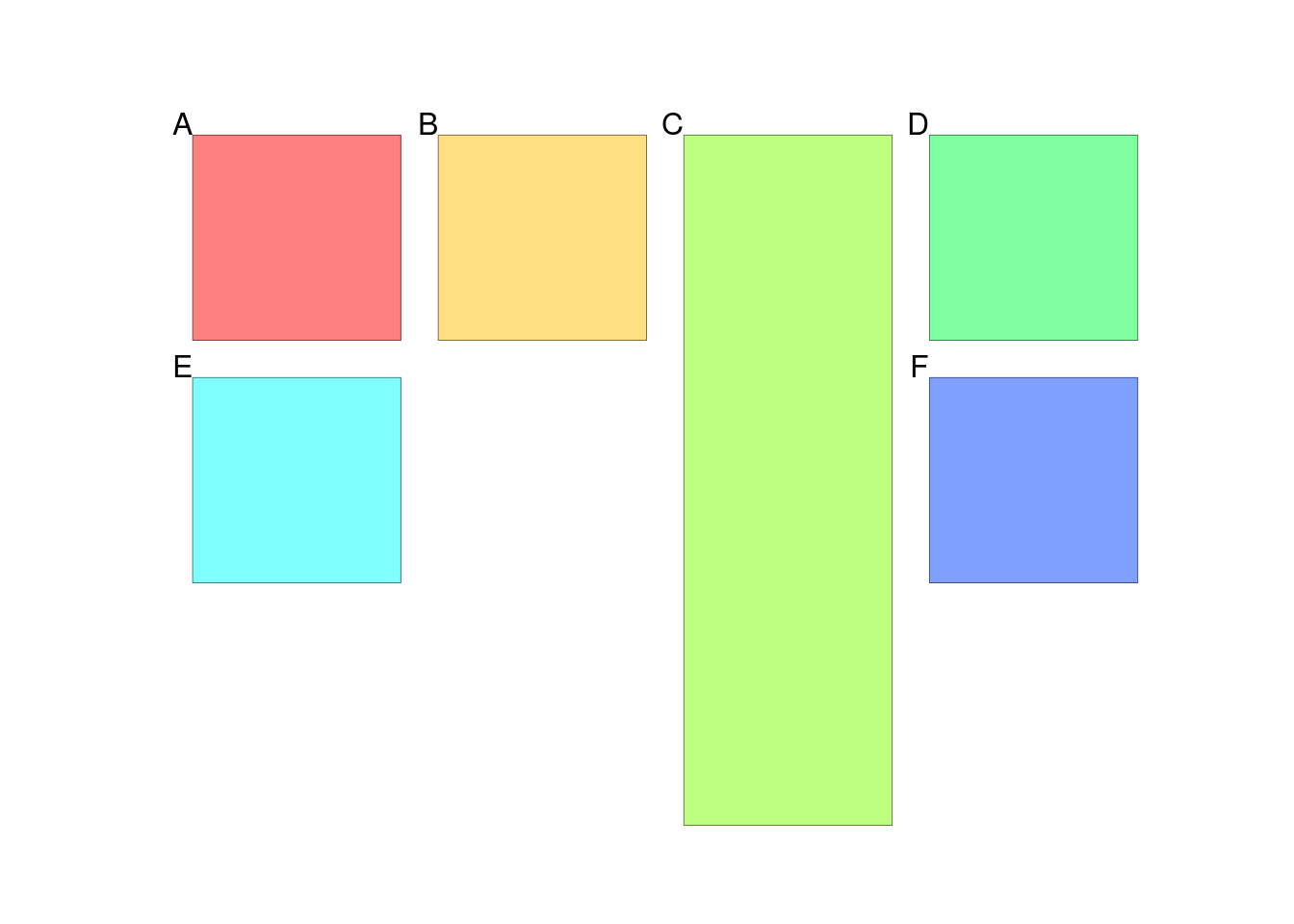Likewise, we can specify the third row, but use automatic positioning for the column.

(figure %<>% fill_panel(rect_grob(), row = 3))
## Setting column to 1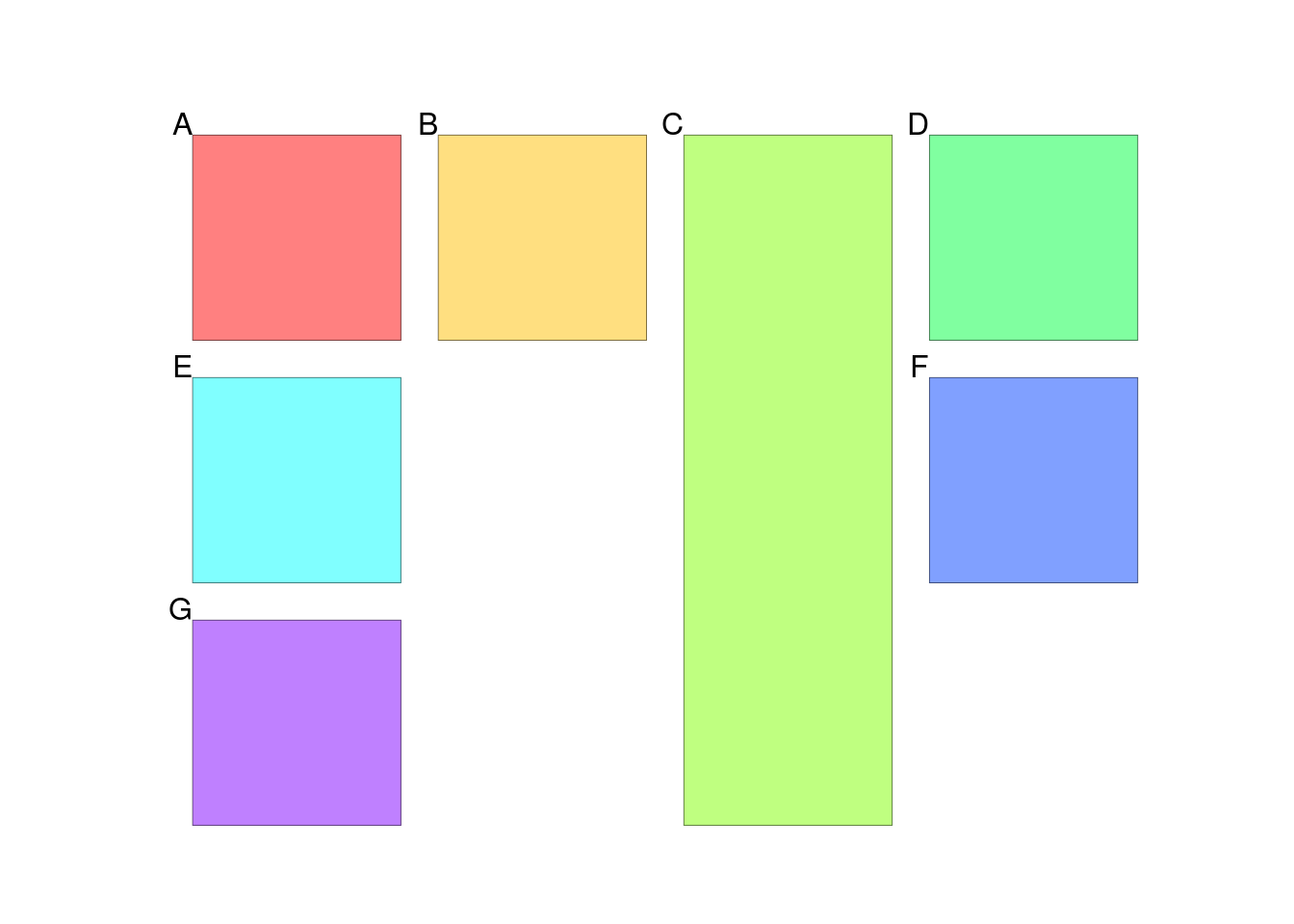If we want to overwrite a panel, by default an error will be thrown. In this case, the panel in the second and third rows, third column have already been filled.

(figure %<>% fill_panel(rect_grob(), column = 2:3, row = 3))
## Error in fill_panel(., rect_grob(), column = 2:3, row = 3): Attempt to use these already filled panels.
##   row col
## 1   3   3

If we really want to overwrite the panel, we can set allow_panel_overwriting = TRUE. The overwritten panels will still be warned about.

(figure %<>% fill_panel(rect_grob(), column = 2:3, row = 3, allow_panel_overwriting = TRUE))
## Warning in fill_panel(., rect_grob(), column = 2:3, row = 3, allow_panel_overwriting = TRUE): Attempt to use these already filled panels.
##   row col
## 1   3   3